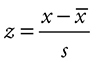# AP Statistics Glossary

Alternative hypothesis—the theory that the researcher hopes to confirm by rejecting the null hypothesis

Association—when some of the variability in one variable can be accounted for by the other

Bar chart—graph in which the frequencies or relative frequencies of categories are displayed with bars

Bimodal—distribution with two most common values; see mode

Binomial distribution—probability distribution for a random variable X in a binomial setting;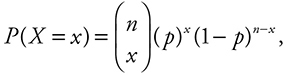where n is the number of independent trials, p is the probability of success on each trial, and x is the count of successes out of the n trials

Binomial setting (experiment)—when each of a fixed number, n, of observations either succeeds or fails, independently, with probability p

Bivariate data—having to do with two variables

Block—a group of experimental units thought to be homogenous with respect to the response variable

Block design—procedure by which experimental units are put into homogeneous groups in an attempt to reduce variability due to the group on the response variable

Blocking—see block design

Boxplot (box-and-whisker plot)—graphical representation of the five-number summary of a dataset. Each value in the five-number summary is located over its corresponding value on a number line. A box is drawn that ranges from Q1 to Q3 and “whiskers” extend to the maximum and minimum values from Q1 and Q3.

Categorical data—data whose values range over categories rather than values. See also qualitative data

Census—attempt to contact every member of a population

Center—the “middle” of a distribution; either the mean or the median

Central limit theorem—theorem that states that the sampling distribution of a sample mean becomes approximately normal when the sample size is large

Chi-square (χ2) goodness-of-fit test—compares a set of observed categorical values to a set of expected values under a set of hypothesized proportions for the categories;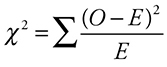Cluster sample—The population is first divided into sections or “clusters.” Then we randomly select an entire cluster, or clusters, and include all of the members of the cluster(s) in the sample.

Coefficient of determination (r2)—measures the proportion of variation in the response variable explained by regression on the explanatory variable

Complement of an event—set of all outcomes in the sample space that are not in the event

Completely randomized design—when all subjects (or experimental units) are randomly assigned to treatments in an experiment

Conditional probability—the probability of one event succeeding given that some other event has already occurred

Confidence interval—an interval that, with a given level of confidence, is likely to contain a population value; (estimate) ± (margin of error)

Confidence level—the probability that the procedure used to construct an interval will generate an interval that does contain the population value

Confounding variable—has an effect on the outcomes of the study but whose effects cannot be separated from those of the treatment variable

Contingency table—see two-way table

Continuous data—data that can be measured, or take on values in an interval; the set of possible values cannot be counted

Continuous random variable—a random variable whose values are continuous data; takes all values in an interval

Control—see statistical control

Convenience sample—sample chosen without any random mechanism; chooses individuals based on ease of selection

Correlation coefficient (r)—measures the strength of the linear relationship between two quantitative variables;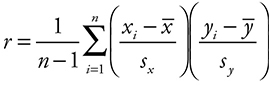Correlation is not causation—just because two variables correlate strongly does not mean that one caused the other

Critical value—values in a distribution that identify certain specified areas of the distribution

Degrees of freedom—number of independent datapoints in a distribution

Density function—a function that is everywhere nonnegative and has a total area equal to 1 underneath it and above the horizontal axis

Descriptive statistics—process of examining data analytically and graphically

Dimension—size of a two-way table; r × c

Discrete data—data that can be counted (possibly infinite) or placed in order

Discrete random variable—random variable whose values are discrete data

Dotplot—graph in which data values are identified as dots placed above their corresponding values on a number line

Empirical Rule (68-95-99.7 Rule)—states that, in a normal distribution, about 68% of the terms are within one standard deviation of the mean, about 95% are within two standard deviations, and about 99.7% are within three standard deviations

Estimate—sample value used to approximate a value of a parameter

Event—in probability, a subset of a sample space; a set of one or more simple outcomes

Expected value—mean value of a discrete random variable

Experiment—study in which a researcher measures the responses to a treatment variable, or variables, imposed and controlled by the researcher

Experimental units—individuals on which experiments are conducted

Explanatory variable—explains changes in response variable; treatment variable; independent variable

Extrapolation—predictions about the value of a variable based on the value of another variable outside the range of measured values

First quartile—the value which identifies the 25th percentile; the value that has at least 25% of the data at or below it and at least 75% of the data at or above it.

Five-number summary—for a dataset, [minimum value, Q1, median, Q3, maximum value]

Geometric setting—independent observations, each of which succeeds or fails with the same probability p; number of trials needed until first success is variable of interest

Histogram—graph in which numerical data are grouped into intervals and the frequencies or relative frequencies within each interval are displayed with bars

Homogeneity of proportions—chi-square hypothesis in which proportions of a categorical variable are tested for homogeneity across two or more populations

Independent events—knowing one event occurs does not change the probability that the other occurs;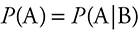Independent variable—see explanatory variable

Inferential statistics—use of sample data to make inferences about populations

Influential observation—observation, usually in the x direction, whose removal would have a marked impact on the slope of the regression line

Interpolation—predictions about the value of a variable based on the value of another variable within the range of measured values

Interquartile range—value of the third quartile minus the value of the first quartile; contains middle 50% of the data

Least-squares regression line—of all possible lines, the line that minimizes the sum of squared errors (residuals) from the line

Line of best fit—see least-squares regression line

Lurking variable—one that has an effect on the outcomes of the study but whose influence was not part of the investigation

Margin of error—measure of uncertainty in the estimate of a parameter; (critical value) · (standard error)

Marginal totals—row and column totals in a two-way table

Matched pairs—experimental units paired by a researcher based on some common characteristic or characteristic

Matched pairs design—experimental design that utilizes each pair as a block; one unit receives one treatment, and the other unit receives the other treatment

Mean—sum of all the values in a dataset divided by the number of values

Median—halfway through an ordered dataset, below and above which lies an equal number of data values; 50th percentile

Mode—most common value in a distribution

Mound-shaped (bell-shaped)—distribution in which data values tend to cluster about the center of the distribution; characteristic of a normal distribution

Mutually exclusive events—events that cannot occur simultaneously; if one occurs, the other doesn’t

Negatively associated—larger values of one variable are associated with smaller values of the other; see association

Nonresponse bias—occurs when subjects selected for a sample do not respond

Normal curve—familiar bell-shaped density curve; symmetric about its mean; defined in terms of its mean and standard deviation;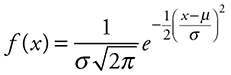Normal distribution—distribution of a random variable X so that P(α < X < b) is the area under the normal curve between α and b

Null hypothesis—hypothesis being tested—usually a statement that there is no effect or difference between treatments; what a researcher wants to disprove to support his/her alternative

Numerical data—see quantitative data

Observational study—when variables of interest are observed and measured but no treatment is imposed in an attempt to influence the response

Observed values—counts of outcomes in an experiment or study; compared with expected values in a chi-square analysis

One-sided alternative—alternative hypothesis that varies from the null in only one direction

One-sided test—used when an alternative hypothesis states that the true value is either less than or greater than the hypothesized value but not both

Outcome—simple events in a probability experiment

Outlier—a data value that is far removed from the general pattern of the data

P(A and B)—probability that both A and B occur; P(A and B) = P(A) · P(A|B)

P(A or B)—probability that either A or B occurs; P(A or B) = P(A) + P(B) – P(A and B)

P-value—probability of getting a sample value at least as extreme as that obtained by chance alone assuming the null hypothesis is true

Parameter—measure that describes a population

Percentile rank—proportion of terms in the distributions less than the value being considered

Placebo—an inactive procedure or treatment

Placebo effect—effect, often positive, attributable to the patient’s expectation that the treatment will have an effect

Point estimate—value based on sample data that represents a likely value for a population parameter

Positively associated—larger values of one variable are associated with larger values of the other; see association

Power of a test—probability of rejecting a null hypothesis against a specific alternative

Probability distribution—identification of the outcomes of a random variable together with the probabilities associated with those outcomes

Probability histogram—histogram for a probability distribution; horizontal axis shows the outcomes, vertical axis shows the probabilities of those outcomes

Probability of an event—relative frequency of the number of ways an event can succeed to the total number of ways it can succeed or fail

Probability sample—sampling technique that uses a random mechanism to select the members of the sample

Proportion—ratio of the count of a particular outcome to the total number of outcomes

Qualitative data—data whose values range over categories rather than values. See also categorical data

Quantitative data—data whose values are numerical

Quartiles—25th, 50th, and 75th percentiles of a dataset

Random phenomenon—unclear how any one trial will turn out, but there is a regular distribution of outcomes in a large number of trials

Random sample—sample in which each member of the sample is chosen by chance and each member of the population has an equal chance to be in the sample

Random variable—numerical outcome of a random phenomenon (random experiment)

Randomization—random assignment of experimental units to treatments

Range—difference between the maximum and minimum values of a dataset

Replication—repetition of each treatment enough times to help control for chance variation

Representative sample—sample that possesses the essential characteristics of the population from which it was taken

Residual—in a regression, the actual value minus the predicted value

Resistant statistic—one whose numerical value is not influenced by extreme values in the dataset

Response bias—bias that stems from respondents’ inaccurate or untruthful response

Response variable—measures the outcome of a study

Robust—when a procedure may still be useful even if the conditions needed to justify it are not completely satisfied

Robust procedure—procedure that still works reasonably well even if the assumptions needed for it are violated; the t-procedures are robust against the assumption of normality as long as there are no outliers or severe skewness.

Sample space—set of all possible mutually exclusive outcomes of a probability experiment

Sample survey—using a sample from a population to obtain responses to questions from individuals

Sampling distribution of a statistic—distribution of all possible values of a statistic for samples of a given size

Sampling frame—list of experimental units from which the sample is selected

Scatterplot—graphical representation of a set of ordered pairs; horizontal axis is first element in the pair, vertical axis is the second

Shape—geometric description of a dataset: mound-shaped; symmetric, uniform; skewed; etc.

Significance level (α)—probability value that, when compared to the P-value, determines whether a finding is statistically significant

Simple random sample (SRS)—sample in which all possible samples of the same size are equally likely to be the sample chosen

Simulation—random imitation of a probabilistic situation

Skewed—distribution that is asymmetrical with data bunched at one end and a tail stretching out in the other

Skewed left (right)—asymmetrical with more of a tail on the left (right) than on the right (left)

Standard deviation—square root of the variance;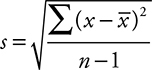Standard error—estimate of population standard deviation based on sample data

Standard normal distribution—normal distribution with a mean of 0 and a standard deviation of 1

Standard normal probability—normal probability calculated from the standard normal distribution

Statistic—measure that describes a sample (e.g., sample mean)

Statistical control—holding constant variables in an experiment that might affect the response but are not one of the treatment variables

Statistically significant—a finding that is unlikely to have occurred by chance

Statistics—science of data

Stemplot (stem-and-leaf plot)—graph in which ordinal data are broken into “stems” and “leaves”; visually similar to a histogram except that all the data are retained

Stratified random sample—groups of interest (strata) chosen in such a way that they appear in approximately the same proportions in the sample as in the population

Subjects—human experimental units

Survey—obtaining responses to questions from individuals

Symmetric—data values distributed equally above and below the center of the distribution

Systematic bias—the mean of the sampling distribution of a statistic does not equal the mean of the population; see unbiased estimate

Systematic sample—probability sample in which one of the first n subjects is chosen at random for the sample and then each nth person after that is chosen for the sample

t-distribution—the distribution with n – 1 degrees of freedom for the t statistic

t statistic—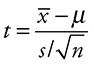Test statistic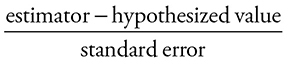Third quartile—the value which identifies the 75th percentile; the value that has at least 75% of the data at or below it and at least 25% of the data at or above it.

Treatment variable—see explanatory variable

Tree diagram—graphical technique for showing all possible outcomes in a probability experiment

Two-sided alternative—alternative hypothesis that can vary from the null in either direction; values much greater than or much less than the null provide evidence against the null

Two-sided test—a hypothesis test with a two-sided alternative

Two-way table—table that lists the outcomes of two categorical variables; the values of one category are given as the row variable, and the values of the other category are given as the column variable; also called a contingency table

Type I error—the error made when a true null hypothesis is rejected

Type II error—the error made when a false null hypothesis is not rejected

Unbiased estimate—mean of the sampling distribution of the estimate equals the parameter being estimated

Undercoverage—some groups in a population are not included in a sample from that population

Uniform—distribution in which all data values have the same frequency of occurrence

Univariate data—having to do with a single variable

Variance—average of the squared deviations from their mean of a set of observations;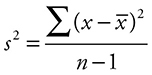Voluntary response bias—bias inherent when people choose to respond to a survey or poll; bias is typically toward opinions of those who feel most strongly

Voluntary response sample—sample in which participants are free to respond or not to a survey or a poll

Wording bias—creation of response bias attributable to the phrasing of a question

z-score—number of standard deviations a term is above or below the mean;